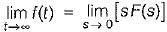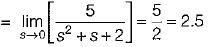Courses

# Transfer Function - 2

## 15 Questions MCQ Test Topicwise Question Bank for Electrical Engineering | Transfer Function - 2

Description
This mock test of Transfer Function - 2 for Electrical Engineering (EE) helps you for every Electrical Engineering (EE) entrance exam. This contains 15 Multiple Choice Questions for Electrical Engineering (EE) Transfer Function - 2 (mcq) to study with solutions a complete question bank. The solved questions answers in this Transfer Function - 2 quiz give you a good mix of easy questions and tough questions. Electrical Engineering (EE) students definitely take this Transfer Function - 2 exercise for a better result in the exam. You can find other Transfer Function - 2 extra questions, long questions & short questions for Electrical Engineering (EE) on EduRev as well by searching above.
QUESTION: 1

### Match List - I (Roots in s-plane) with List - II (Corresponding impulse response) and select the correct answer using the codes given below the lists: List - I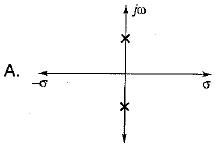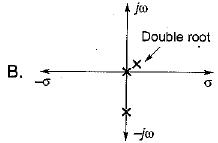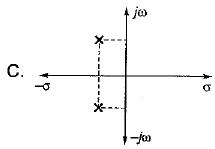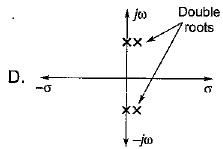List - II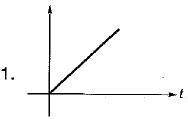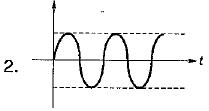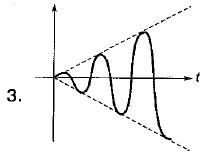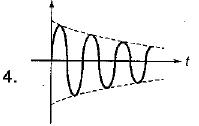Codes: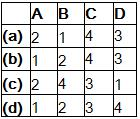Solution:

For A: Two conjugate poles on imaginary axis i.e. it’s impulse response = sin at (marginally stable).
For B: Two poles at origin i.e. impulse response = t u(t) (unstable system).
For C: Two complex conjugate poles with negative real parts. So, its impulse response = er-at sin bt (stable system).
For D: Two imaginary complex double roots. So, impulse response = t sin bt. (unstable system).

QUESTION: 2

Solution:
QUESTION: 3

### The transfer function is applicable to

Solution:

Transfer function is applicable to only linear and time-variant systems.

QUESTION: 4

The DC gain of the system represented by the following transfer function is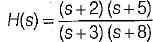Solution:

Given transfer function is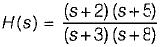Converting above transfer function in time-constant form, we get: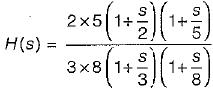Hence, dc gain is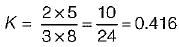QUESTION: 5

The unit impulse response of a control system is given by c(t) = -te-t + e-t. Its transfer function is

Solution:

Given, c(t) = -te-t + e-t (impulse response)
We know that,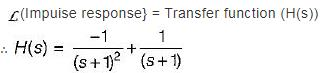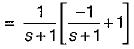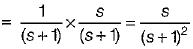QUESTION: 6

The impulse response of an initially relaxed linear system is e-3t u(t). To produce a response of te-3t u(t), the input must be equal to

Solution:

We know that, impulse response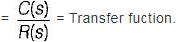For given system,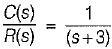Now, c(t) = t e-3t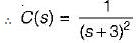So, R{s) = C(s) x (s + 3)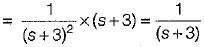or, r(t) = Required input = e-3t u(t)

QUESTION: 7

A linear time invariant system, initially at rest when subjected to a unit step input gave response c(t) = te-t(t ≥ 0). The transfer function of the system is

Solution:

Given, c(t) = te-t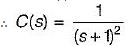Now, r(t) = u(t) (unit step input)
∴ R(s) = 1/s
So,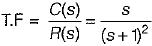QUESTION: 8

A linear time invariant system having input r(t) and output y(t) is represented by the differential equation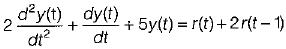The transfer function of the given system is represented as

Solution:

Taking Laplace transform of the given differential equation on both sides, we get
2s2 Y(s) + sY(s) + 5 Y(s) = R(s) + 2e-s R(s)
or,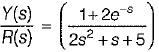QUESTION: 9

The open loop transfer function of a system is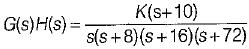A closed loop pole will be located at s = -12
when the value of K is

Solution: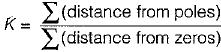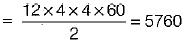QUESTION: 10

The singularities of a function are the points in the s-plane at which the function or its derivates

Solution:
QUESTION: 11

Assertion (A): The final value theorem cannot be applied to a function given by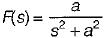Reason (R): The function s F(s) has two poles on the imaginary axis of s-plane.

Solution:

The final value theorem is valid only if sF(s) does not has any poles on the jω axis and in the right half of the s-plane. Hence, both A and R are true and R is a correct explanation of A.

QUESTION: 12

When two time constant elements are cascaded non-interactively then, the overall transfer function of such an arrangement

Solution:
QUESTION: 13

The unit impulse response of a unity feedback control svstem whose Open Iood transfer function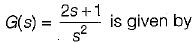Solution:

Given, O.L.T.F.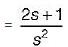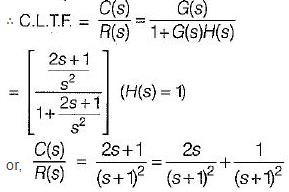For unit impulse response, R(s) = 1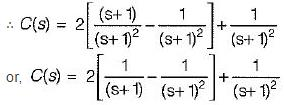or, c(t) = 2(e-t - te-t) + te-t
= 2e-t - te-t = (2- t) e-t
Thus, impulse response, c(t) = (2 - t) e-t u(t)

QUESTION: 14

The impulse response of a linear time invariant system is a unit step function. The transfer function of this system would be

Solution:

Impulse response,
c(t) = u(t)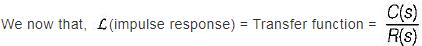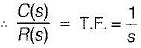QUESTION: 15

Consider the function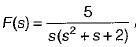where F(s) is the Laplace transform of f(t)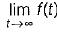is equal to

Solution:

Using final value theorem